# Polygons

Here you will learn about polygons, including types of polygons and how to classify them.

Students first learn about polygons as part of their work in geometry in 2nd and 3rd grade.

## What are polygons?

Polygons are enclosed 2D shapes with straight sides. Two sides of a polygon meet at a vertex. 2D polygons are flat, meaning they are plane figures.

Here is an example of a polygon:

Polygons have interior angles and exterior angles.

### Types of polygons

The table gives the names of various polygon with their number of sides.

PolygonShapeNumber of sides
Triangle
3
4
Pentagon
5
Hexagon
6
Heptagon
7
Octagon
8
Nonagon
9
Decagon
10
Hendecagon
11
Dodecagon
12

### How to classify polygons

Polygons can be regular or irregular.

Regular polygons:Irregular polygons:

● All the sides are the same length.
● All angles are the same size.

A square is a regular quadrilateral.● All the sides are not the same length.
● All the angles are not the same side.

A trapezoid is an irregular quadrilateral.

Polygons can be convex or concave.

Convex polygons:Concave polygons:

● Have interior angles that are acute,
obtuse or right.

● Have at least one interior angle that
is greater than 180^{\circ}.

### What are polygons?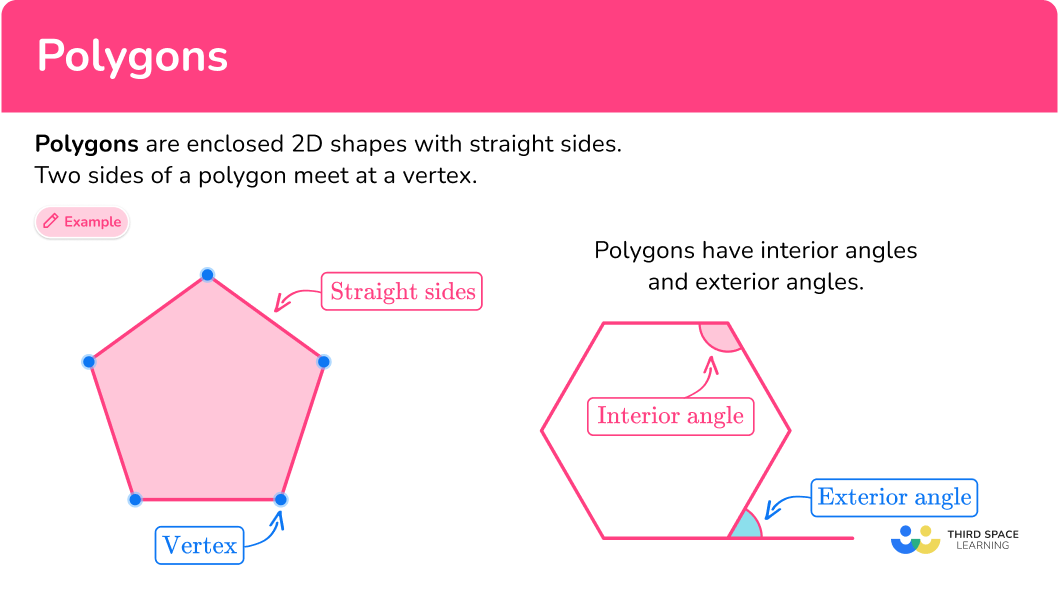## Common Core State Standards

How does this apply to second and third grade math?

• Grade 2 – Geometry (2.G.A.1):
Recognize and draw shapes having specified attributes, such as a given number of angles or a given number of equal faces. Identify triangles, quadrilaterals, pentagons, hexagons, and cubes.

• Grade 3 – Geometry (3.G.A.1):
Understand that shapes in different categories (example, rhombuses, rectangles, and others) may share attributes (example, having four sides), and that the shared attributes can define a larger category (example, quadrilaterals).

Recognize rhombuses, rectangles, and squares as examples of quadrilaterals, and draw examples of quadrilaterals that do not belong to any of these subcategories.

## How to classify a polygon

In order to classify a polygon:

1. State the number of sides.
2. Recall the definition.
3. Classify the polygon.

## Polygons examples

### Example 1: classify the shape by sides and regular or irregular

Name the type of polygon.

1. State the number of sides.

The polygon has 6 sides.

2Recall the definition.

A regular polygon has sides that are the same length and angles that are the same size.

An irregular polygon has sides that are not the same length and angles that are the same size.

The sides are the same size which means they are equal (congruent).

The angles are the same measure which means they are equal (congruent).

3Classify the polygon.

The polygon is a regular hexagon.

### Example 2: classify the shape by sides and regular or irregular

Name the type of polygon.

A regular polygon has sides that are the same length and angles that are the same size.

An irregular polygon has sides that are not the same length and angles that are the same size.

The angles are all equal because they are all 90^{\circ} .

The sides are not the same measure, which means they are not equal (congruent).

The polygon appears to be a rectangle, which is an irregular quadrilateral.

### Example 3: classify the shape by sides and regular or irregular

Name the type of polygon.

A regular polygon has sides that are the same length and angles that are the same size.

An irregular polygon has sides that are not the same length and angles that are the same size.

The sides are different lengths, so they are not equal (congruent).

The angles are different measures, so they are not equal (congruent).

The polygon is an irregular pentagon.

### Example 4: classify the shape by sides and regular or irregular

Name the type of polygon.

A regular polygon has sides that are the same length and angles that are the same size.

An irregular polygon has sides that are not the same length and angles that are the same size.

The sides are the same length (congruent) and the angles are the same measure (congruent).

The polygon is a regular octagon.

### Example 5: classify the shape by sides and regular or irregular

Here is a picture of Mary’s fenced in yard. Describe the polygon it makes.

4 sided polygon.

A regular polygon has sides that are the same length and angles that are the same size.

An irregular polygon has sides that are not the same length and angles that are the same size.

The angels are all different sizes.

The sides are all different sizes.

Mary’s yard is an irregular quadrilateral.

### Example 6: classify the shape by sides and regular or irregular

Describe the polygon that is made by the baseball field.

6 sides

A regular polygon has sides that are the same length and angles that are the same size.

An irregular polygon has sides that are not the same length and angles that are the same size.

The angles are not all equal to each other.

The sides are not all equal to each other.

The baseball field is an irregular hexagon.

### Teaching tips for polygons

• Incorporate learning activities that provide students with the opportunity to draw out the polygons so that they can formulate their own understanding.

• Include cross curricular art activities so students can be creative with polygons.

• Provide opportunities for students to see polygons in a real-life setting through architecture.

• Although worksheets have their place in the classroom, having students practice skills through interactive digital platforms and game playing is more motivating and engaging.

### Easy mistakes to make

• Thinking that polygons with any equal angles and sides are regular
Regular polygons have all sides and angles that are the same size and equal.
For example,
A square is a regular polygon, and a trapezoid is an irregular polygon, even though the trapezoid has some angles that are the same size and some sides that are the same size.

### Practice polygons questions

1. Select the polygon that is a hexagon.Hexagons are polygons with 6 sides. This was the only polygon choice with 6 sides.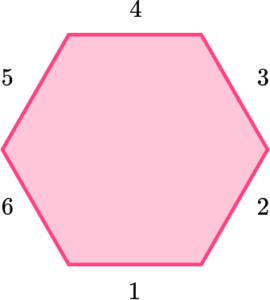2. Select the polygon that is an octagon.An octagon is a polygon that has 8 sides.

This is the only polygon with 8 sides.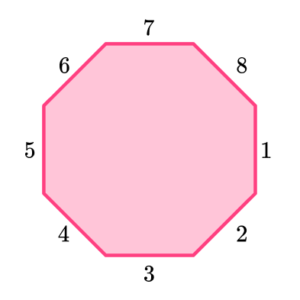3. Which polygon is a regular pentagon?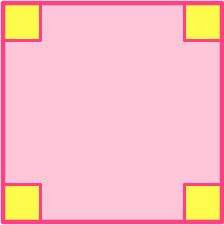A regular pentagon has 5 sides that are the same size and 5 angles that are the same size.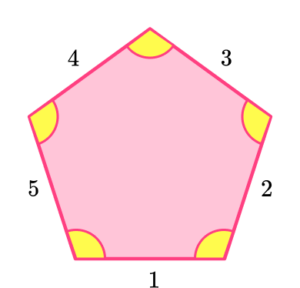4. Which polygon is a regular quadrilateral?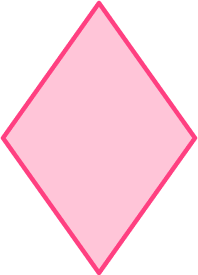A regular quadrilateral is a 4 sided polygon that has 4 equal sides and 4 equal angles. A square is a regular quadrilateral.5. Which polygon best describes this field?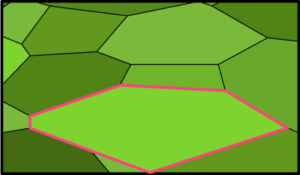Regular pentagonRegular hexagonIrregular pentagonIrregular hexagonThe field has six sides.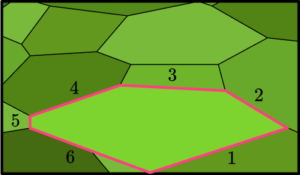Six sided polygons are hexagons. The hexagon is also an irregular polygon. So the field is an irregular hexagon.

6. Which polygon best describes the yard?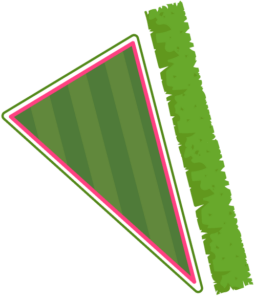Irregular triangleEquilateral triangleRhombusThe yard has 3 sides so it is a triangle. The sides angles are not all the same size.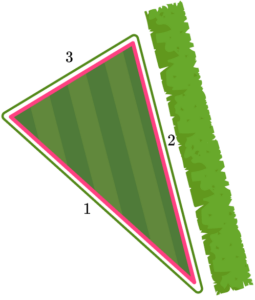## Polygons FAQs

What is a pentagram?

A pentagram is a star which can be seen as a complex polygon made up of one pentagon and 5 triangles.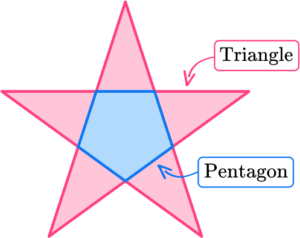What are the diagonals of polygons?

Diagonals are line segments connecting non-consecutive vertices of the polygons. The example below shows a parallelogram with one of its diagonals. There are several geometry theorems that you will learn in high school that describe properties of diagonals of certain polygons.Is there a name for every polygon?

There are some simple names for polygons like pentagons, hexagons, etc., but not all polygons have simple names. The term n -gon is used for polygons without simple names where n represents the number of sides (n sides). For example, a 22 -gon is a polygon with 22 sides. It’s proper name is an icosikaidigon!

## Still stuck?

At Third Space Learning, we specialize in helping teachers and school leaders to provide personalized math support for more of their students through high-quality, online one-on-one math tutoring delivered by subject experts.

Each week, our tutors support thousands of students who are at risk of not meeting their grade-level expectations, and help accelerate their progress and boost their confidence.